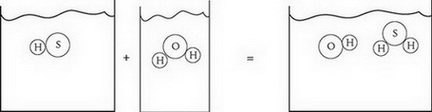# AP Chemistry Practice Test 7

### Test Information12 questions18 minutes

1. The average mass, in grams, of one mole of carbon atoms is equal to

2.The diagram above best represents which type of reaction?

3. Which of the following is true for all bases?

`Questions 4-6 refer to the following information.14H+(aq) + Cr2O72-(aq) + 3Ni(s) →2Cr3+(aq) + 3Ni2+(aq) + 7H2O(l)In the above reaction, a piece of solid nickel is added to a solution of potassium dichromate.`

4. Which species is being oxidized and which is being reduced?

Oxidized    Reduced

5. How many moles of electrons are transferred when 1 mole of potassium dichromate is mixed with 3 mol of nickel?

6. How does the pH of the solution change as the reaction progresses?

7. A sample of an unknown chloride compound was dissolved in water, and then titrated with excess Pb(NO3)2 to create a precipitate. After drying, it is determined there are 0.0050 mol of precipitate present. What mass of chloride is present in the original sample?

8. A photoelectron spectra for which of the following atoms would show peaks at exactly three different binding energies?

9. The bond length between any two nonmetal atoms is achieved under which of the following conditions?

10. Hydrogen fluoride, HF, is a liquid at 15°C. All other hydrogen halides (represented by HX, where X is any other halogen) are gases at the same temperature. Why?

11.

 Initial pH pH after NaOH addition Acid 1 3.0 3.5 Acid 2 3.0 5.0

Two different acids with identical pH are placed in separate beakers. Identical portions of NaOH are added to each beaker, and the resulting pH is indicated in the table above. What can be determined about the strength of each acid?

12. A stock solution of 12.0 M sulfuric acid is made available. What is the best procedure to make up 100. mL of 4.0 M sulfuric acid using the stock solution and water prior to mixing?